Search IntMath
Close

450+ Math Lessons written by Math Professors and Teachers

5 Million+ Students Helped Each Year

1200+ Articles Written by Math Educators and Enthusiasts

Simplifying and Teaching Math for Over 23 Years

# IntMath Newsletter: Learning context, math notation history

By Murray Bourne, 28 May 2013

28 May 2013

1. Conditional stupidity
2. Resource: History of Mathematical Notation
3. IntMath polls
4. Math puzzles
5. Final thought: Listening

As mentioned in previous Newsletters, I'm snowed under with projects at the moment. I hope the second half of the year is a bit more relaxed so the Newsletter can come out more regularly!

## 1. Conditional stupidity

You know how sometimes you feel confident to do something, while in other situations you feel totally lost trying to do the same thing?

My own example is that horrible situation sports teachers constantly used to put us in. They wanted us to play some team sport and so 2 teams are needed. Two "captains" are appointed, and they start choosing from the total group of students. The "best" ones are chosen first, of course. What captain wants to deliberately lose?

And finally they'd get to the "leftovers" - the students neither side wants because of their known lack of prowess with footballs or whatever. Yep, and that used to include me.

The game would start. Everyone on the other side knew who were the weaker ones on our side, so would set up situations where they knew they could get easy points. Of course, us weaker ones would try our hardest to play well, but would inevitably fail due to the stress of trying to perform but with no support from out team mates (and a feeling of rejection from the other side).

In a different setting with supportive team mates, we could perform adequately (not brilliantly, but at least better). The difference was the social context in which we operated.

What's this got to do with math?

Our learning is always affected by the social climate surrounding us. "Stereotype threat" has a big influence on how well we perform in any situation.

Tell a group of girls that "women perform worse than men on math tests", then give them a math test, and they perform worse than another group of girls who are told they doing an experiment and to "do your best". Why? Because the girls in the first group are scared to "let down the team" and prove the stereotype correct.

The heightened anxiety is self-fulfilling, and they perform worse.

In Fragility of Intelligence, Annie Murphy Paul outlines many similar examples where we "sometimes feel smart and sometimes feel dumb."

One such example is...

White male math and engineering majors who received high scores on the math portion of the SAT did worse on a math test when told that the experiment was intended to investigate “why Asians appear to outperform other students on tests of math ability.”

In another example, we learn an unsafe environment leads to a drop in performance.

In a study conducted on the troubled South Side of Chicago, for example, students whose neighborhoods had been the site of a homicide within the previous two weeks scored half a standard deviation lower on a test of intelligence.

I think in many math classes the concentration is too much on "the content", and not enough on "the context".

It's worth reading all of Fragility of Intelligence.

## 2. Resource: History of Mathematical Notation

Florian Cajori (1859 - 1930) was a well-known mathematical historian. Among his publications were A History of Mathematics, A History of the Logarithmic Slide Rule and Allied Instruments, and William Oughtred: a Great Seventeenth-century Teacher of Mathematics.

His research bordered on the obsessive, and his works are regarded as classics in the field.Florian Cajori [Image source]

His books are now out of copyright and have passed into the public domain (since he died over 70 years ago), so are freely available.

For quite some time now I've had an interest in the ways poor math notation gets in the way of students' understanding of math concepts.

Cajori's A History of Mathematical Notations sheds light on some of the interesting quirks of notation throughout history.

It's available in several formats, including an online reader, PDF, EPUB, Kindle and full text, from this link:

In Cajori's book, we discover that in the 16th century it was quite common to use the division sign (÷) to mean subtraction (p. 240).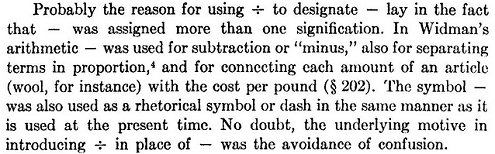Different meanings for the symbol — [Image source]

I've never liked the division sign. It often looks too much like a plus, depending on the font used, and especially if we are using a printer with enthusiastic nozzles. The forward slash (/) as used in many countries is probably better, but still open to some confusion, especially with the vertical bar (|) used in modular arithmetic and for absolute value.

And just to pick up Cajori's last point, most math notation is adopted for convenience, rather than reducing confusion. For example, algebra makes heavy use of letters in place of numbers. It's convenient, but it sure confuses a lot of people.

We also read (p. 328) about the controversy regarding use of the comma (,) rather than the dot (.) for writing decimal numbers. In fact, during the early 1700s, there were 8 different ways mathematicians would write decimal numbers, including the use of dots, commas, wedges, and subscripts: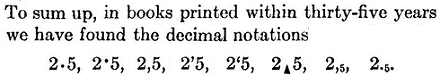Different ways of writing "two point five" [Image source]

We need to remember that such symbols were not meant for mass consumption. At the time, the majority of the population received no education at all, so notation could be fairly fluid. As long as the close group of friends or collaborators knew what your inconsistent notation meant, it was OK.

However, now that education of the masses is more the norm, and most students study mathematics, I feel we should tighten math notation and make it more consistent.

Cajori's book helps us to understand why we use such a range of math notation for the same thing.

The link again: A History of Mathematical Notations

## 3. IntMath Polls

In math class, which is the most common delivery method for the math questions you need to do?

Worksheets: 34%

Text book: 29%

On the board: 20%

Online: 15%

Other: 2%

It seems worksheets are a very common way to deliver math questions. I encourage those of you who are math teachers to explore better ways! Here's some food for thought: 5 Reasons Why Math Worksheets Don’t Work (link no longer available)

Latest poll: As we near the summer holidays in the northern hemisphere, the current poll asks about your feelings concerning the length of summer holidays. You can respond on any (inner) page of IntMath.

## 4. Math puzzles

The puzzle in the last IntMath Newsletter involved finding how many unit cubes could fit into a sphere of radius 3 units.

The correct answer (56) with reasoning was given by John Rowland, Dave Marain, Tim Slason and Hossein (who provided a neat Matlab code-based solution).

One way of approaching this problem is to consider horizontal slices at z = 0, z = 1 and z = 2 (where the x- and y-axes are taken as horizontal, and the z-axis is vertical).

Here is the view when looking from the top when we slice at z = 1. The outer circle is the "equator" of our sphere (z = 0 slice, radius 3), and the inner circle, with radius √8 ≈ 2.828, is the circle that results from the z = 1 slice.

We see we can (just) fit 16 cubes under that z = 1 slice. (The diagonal of a 2 × 2 square is √8 units.)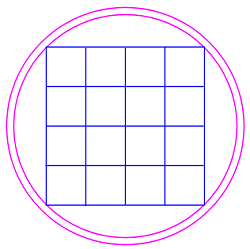Sphere with slices at z = 0, 1, and unit cubes

Next we slice at z = 2. The innermost circle now in our diagram has radius √5 ≈ 2.236. Between the z = 1 slice and the z = 2 slice, we can fit 12 complete unit cubes, as shown. (The diagonal of a 2 × 1 rectangle is √5 units.)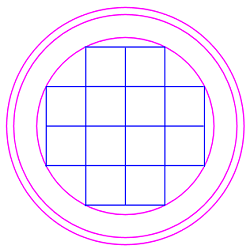Sphere with slices at z = 0, 1 and 2, and unit cubes between 1 and 2

We can't fit any cubes above that. So that's 28 cubes in the top half, giving us 56 altogether.

Here's a (stylized) picture of all 56 cubes, courtesy of John Rowland.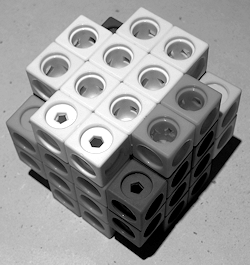[Image source: SofARMaths]

Here's a 3-D view of our sphere, with the 28 unit cubes nestled under the z = 1 and z = 2 slices.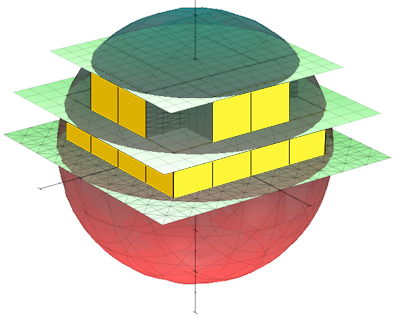Sphere with slices at z = 0, 1 and 2, and unit cubes

#### New puzzle

Function f(x) is discontinuous at x = 2, as shown.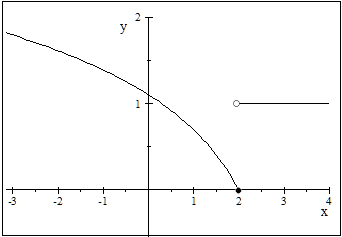Function f(x).

Function g(x) is also discontinuous at x = 2, as shown.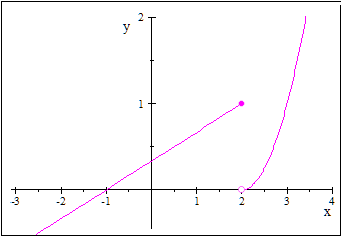Function g(x).

What is the value of:

(a) The limit of the sum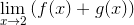(b) The limit of the product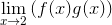## 5. Final thought: Listening

These days I spend quite a bit of time in classrooms as an observer. I actually spend less time watching the teacher than I do observing how the students react to what is going on. In many classrooms I notice teachers love to talk. But actually, the best way to engage students - especially math students - is to listen.

The following quote is from Donald Trump, the successful real estate millionaire. I think it's a saying that could apply equally as well to math teachers and math students.

Listen. Don't just wait to talk. [Donald Trump]

Until next time, enjoy whatever you learn.

1. gagangc says:

a.limit of sum doesn't exist(not defined).
b.limit of product as x tends to 2 is equal to 0.

2. SACHIN says:

I am very much agree with the statement listening in the final thought...Nice words

3. Nosipho Mathebula says:

1.The limit does not exist since both functions have jumps.And according to the limit laws,the sum of a limit is the limit of each function in the sum.Thus if the limit of f(x) does not exist since the limit from the left is not equal to the limit on the right(due to the jump),same for g(x),the sum of their limits would not exist.

2.The limit does not exist.Same reasoning as above.The limit of the product does not exist since each of the limits do not exist.

4. Fátima says:

About the Conditional stupidity section: how important is to provide a safe and a comfortable environment to the students. I really appreciate to all those teachers who try their best to inspirit young people.

5. abhijit says:

1) limx->2- (f(x)+g(x)) = 0 +1 =1

2) limx->2+ (f(x)+g(x)) = 1 +0 =1

3) limx->2- (f(x)Xg(x)) = 0

4) limx->2+ (f(x)Xg(x)) = 0

5) limx->2 DO NOT Exist

6. Ahmed M. Nageeb says:

the limit of the sum will be 2 from both sides so the limit of the sum exists and = 2
the limit of the product will be 0 from both sides so it exists and the limit of the product = 0

7. Guido says:

First examine both the left and right limit of the two functions.
LIM(from -inf->2)of f(X)= 0 ; LIM(from +inf->2)of f(X)= 1
LIM(from -inf->2)of g(X)= 1 ; LIM(from +inf->2)of g(X)= 0

As a result of the properties of limits:
LIM{f(X)+g(X)}=LIM{f(X)}+LIM{g(X)}=0+1=1 or 1+0=1
LIM{f(X)*g(X)}=LIM{f(X)}*LIM{g(X)}=0*1=0 or 1*0=0

So the results of the left and right limits are the same,
The limit of the sum of the functions is 1 and
the limit of the product of the functions is 0

8. liuting says:

The second part is very intersting and important Historical knowledge about math notation.

9. Sachin Munshi says:

I think both limits do not exist. The limit laws for sums and products of functions are

lim(x->a) [f(x)+g(x)]= lim(x->a) f(x) + lim(x->a) g(x), and
lim(x->a) [f(x)g(x)]= lim(x->a) f(x) * lim(x->a) g(x).

The limit of f does not exist, since both one-sided limits are not equal, and the same for g. Hence, the limits of f+g and fg do not exist. I hope this makes sense.

10. Douglas J. Gerrard says:

The limit of the sum is 1 and that of the product is zero. This is because as the limits are approached from the left or the right, they both prevail.

11. Thoughts This Week… | Mathematics, Learning and Web 2.0 says:

[...] always very clearly laid out and I always find something of interest. Recently he talked about the History of Mathematical Notation (see Item 2), note the now free download. See this page for more useful [...]

12. hamid zandi says:

a)1
b)0

13. Tim Slason says:

I may be missing something vital in the definition of limits, but it would appear that (a) would be 1, and (b) would be zero.

(a) is lim(0+1) from the left and lim(1+0) from the right, since those are both 1 the limit exists.

(b) is lim(0*1) and lim(1*0) respectively as well, leading me to my conclusion.

### Comment Preview

HTML: You can use simple tags like <b>, <a href="...">, etc.

To enter math, you can can either:

1. Use simple calculator-like input in the following format (surround your math in backticks, or qq on tablet or phone):
a^2 = sqrt(b^2 + c^2)
(See more on ASCIIMath syntax); or
2. Use simple LaTeX in the following format. Surround your math with $$ and $$.
$$\int g dx = \sqrt{\frac{a}{b}}$$
(This is standard simple LaTeX.)

NOTE: You can mix both types of math entry in your comment.